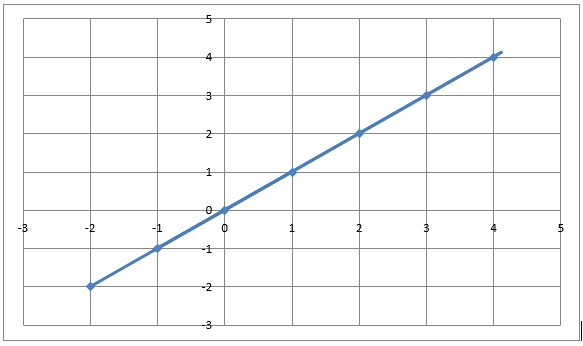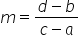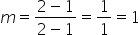Maths-
General
Easy

Question

# Sketch an example of a linear function in graph form.Hint:

## The correct answer is: y=x

### We shall draw a straight line through the origin as shown below.We will now derive the equation of this line in slope intercept form.The slope intercept form isy = mx + cWhere m is the slope and c is the y intercept.From the graph, we can see that the line cuts the y axis at 0So, c = 0The slope between two points (a, b) and (c, d) is given byWe take any two points from the graph to be(a, b) = (1,1) and (c, d) = (2, 2)Calculating the slope, we havePutting the value of m and c in the equation, we get                                  y = 1.xThat is                                   y = xThis is a linear equation.

We can take any straight line in the xy plane and derive its equation in the above method; we will get a linear function. We can check this by calculating the slope between all the points. If the slope is constant everywhere, then it is linear.#### With Turito Foundation.#### Get an Expert Advice From Turito.